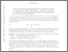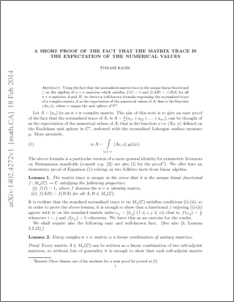# A short proof of the fact that the matrix trace is the expectation of the numerical values

Kania, Tomasz (2015) A short proof of the fact that the matrix trace is the expectation of the numerical values. American Mathematical Monthly, 122 (8). pp. 782-783. ISSN 0002-9890Preview
PDF (1402.4272v1)
1402.4272v1.pdf - Accepted Version

## Abstract

Using the fact that the normalised matrix trace is the unique linear functional $f$ on the algebra of $n\times n$ matrices which satisfies $f(I)=1$ and $f(AB)=f(BA)$ for all $n\times n$ matrices $A$ and $B$, we derive a well-known formula expressing the normalised trace of a complex matrix $A$ as the expectation of the numerical values of $A$; that is the function $\langle Ax,x\rangle$, where $x$ ranges the unit sphere of $\mathbb{C}^n$.

Item Type:
Journal Article
Journal or Publication Title:
American Mathematical Monthly
Uncontrolled Keywords:
/dk/atira/pure/subjectarea/asjc/2600
Subjects:
Departments:
ID Code:
73792
Deposited By:
Deposited On:
18 Jun 2015 05:45
Refereed?:
Yes
Published?:
Published